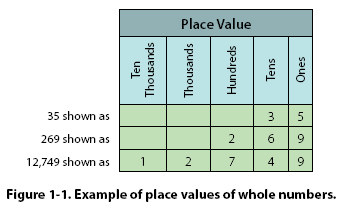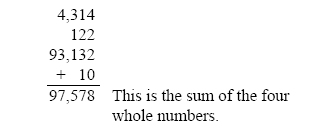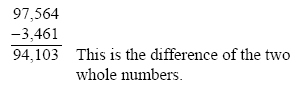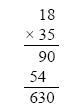CHAPTER 1. Mathematics Mathematics in Aviation Maintenance Mathematics is woven into many areas of everyday life. Performing mathematical calculations with success requires an understanding of the correct methods and procedures, and practice and review of these principles. Mathematics may be thought of as a set of tools. The aviation mechanic will need these tools to successfully complete the maintenance, repair, installation, or certification of aircraft equipment. Many examples of using mathematical principles by the aviation mechanic are available. Tolerances in turbine engine components are critical, making it necessary to measure within a ten-thousandth of an inch. Because of these close tolerances, it is important that the aviation mechanic be able to make accurate measurements and mathematical calculations. An aviation mechanic working on aircraft fuel systems will also use mathematical principles to calculate volumes and capacities of fuel tanks. The use of fractions and surface area calculations are required to perform sheet metal repair on aircraft structures. Whole NumbersWhole numbers are the numbers 0, 1, 2, 3, 4, 5, and so on. Addition of Whole Numbers Addition is the process in which the value of one number is added to the value of another. The result is called the sum. When working with whole numbers, it is important to understand the principle of the place value. The place value in a whole number is the value of the position of the digit within the number. For example, in the number 512, the 5 is in the hundreds column, the 1 is in the tens column, and the 2 is in the ones column. The place values of three whole numbers are shown in Figure 1-1.   When adding several whole numbers, such as 4,314, 122, 93,132, and 10, align them into columns according to place value and then add.Subtraction of Whole Numbers Subtraction is the process in which the value of one number is taken from the value of another. The answer is called the difference. When subtracting two whole numbers, such as 3,461 from 97,564, align them into columns according to place value and then subtract.Multiplication of Whole Numbers Multiplication is the process of repeated addition. For example, 4 x 3 is the same as 4 + 4 + 4. The result is called the product. Example: How many hydraulic system filters are in the supply room if there are 35 cartons and each carton contains 18 filters?Therefore, there are 630 filters in the supply room.
 ©AvStop Online Magazine                                                                                                                                                      Contact Us              Return To Books